Search
•Shalmali

# Newtonian and Non- Newtonian Fluids

What are viscosity and its types?Viscosity is one of the properties of the fluid. And yes! Newton has to say something about it too!

Let's see what he stated and how the viscosity of fluids works!

## Viscosity

Viscosity can be simply defined as the measure of a fluid's resistance to flow.

When fluid is in motion or force is applied against the fluid to flow, there is resistance to the flow. The fluid molecules resist the motion due to cohesive (intermolecular) forces between themselves. The fluid molecules near the plate or pipe do not move due to inertia, in turn, take energy from the adjacent molecules and slow them down too! Sounds like a crab right? Click here to understand it in detail.

### Dynamic / Absolute Viscosity: Newtonian Fluids

Newton’s law of viscosity defines the relationship between the shear stress and shear rate of a fluid subjected to mechanical stress.

The ratio of shear stress to shear rate is a constant, for a given temperature and pressure, and is defined as the viscosity or coefficient of viscosity. 

The best example for this type of fluid is water and air, other examples being alcohol, glycerol, etc. Dynamic Viscosity, coefficient of viscosity, or viscosity is the same with SI units, Pa-s (Pascal second), and Poise and denoted by μ (mu).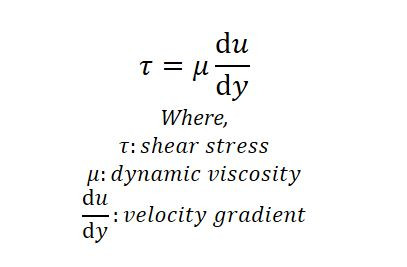Dynamic Viscosity

1 poise = 100 centipoise = 1 g/cm.s = 0.1 Pa s

1 centipoise = 1 mPa.s

As you can see in the graph above, the curve for Newtonian fluids is linear which passes through the center of the graph. The viscosity is independent of the shear rate.

Newton’s observations led him to believe that a fluid’s viscosity was simply a function of shear stress and temperature — and that it would remain unchanged no matter the shear rate. But... obviously science never fails to surprise us!

### Kinematic Viscosity: Non-Newtonian Fluids

Kinematic viscosity is the dynamic viscosity divided by density.

SI unit of kinematic viscosity is m^2/s and is denoted by v (new).Kinematic viscosity

Here, viscosity (ratio of shear stress to shear rate) is not constant and is dependent on the shear rate.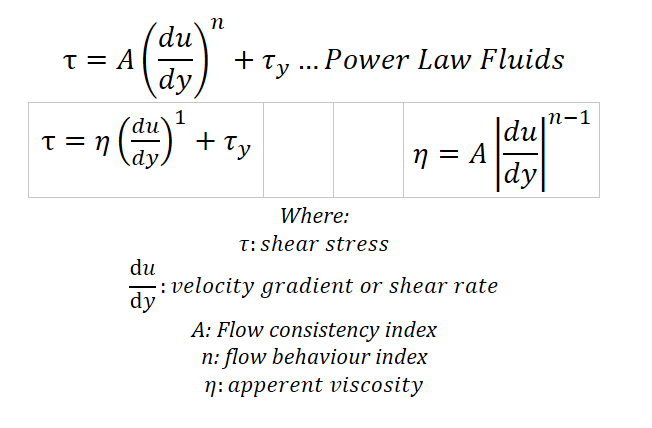Power Law

The curve of the fluid in the graph depends on the flow behavior index (n).

## Types of fluids

1. Newtonian Fluids

2. Non-Newtonian Fluids

### Dilatant or Shear Thickening fluids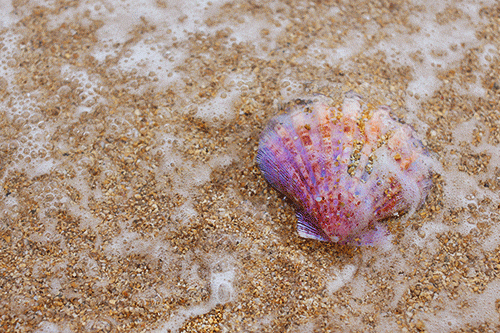It is a type of material whose viscosity increases as the rate of shear strain increases. In simple words, viscosity increases as stress increases.

Examples: Corn starch and water (oobleck), sand in water, etc.

As more force is applied to the oobleck, stress increases, and in turn, the viscosity also increases.

Just imagine diving into a pool of dilatant! It hurts!

Watch this video of placing oobleck on speakers!!!

Application: Body Armour

Military applications like body armor for flexible body movements and sudden attacks, like bullets or knives.

### Pseudoplastic or Shear Thinning fluidsThis is a more common type of fluid than dilatant, which occurs naturally. It is a type of material that is opposite of dilatant, which means the viscosity decreases as the shear rate increases.

Examples: Quicksand, blood, milk, paints, nail polish, ketchup, etc.

Ketchup is an easy example to visualize, the more force you apply, the faster it moves. Or imagine running on a quicksand!Flow behavior index for different fluids

### Rheopectic Fluids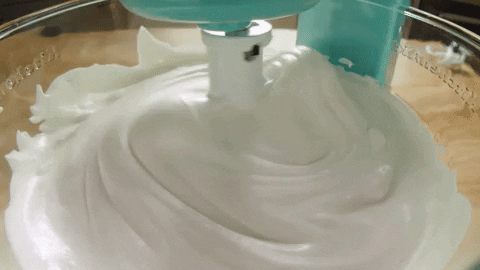It is a type of material, whose viscosity increases as stress with respect to time increases. It is a time-dependent fluid. Rheopectic fluids should not be confused by dilatant fluid. A lot of research is going on, on these fluids for military purposes.

Examples: Printer Ink, whipped cream, etc

The longer you whip the cream, the thicker it gets. (But of course, it gets thick up to a certain point!)

### Thixotropic Fluids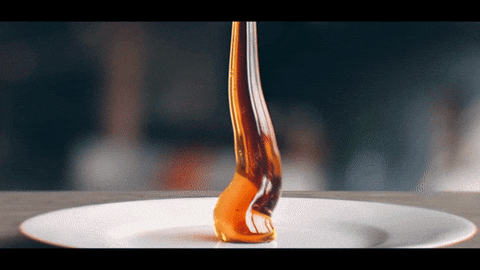It is a type of fluid whose viscosity decreases as stress with respect to time increases. Similar to Rheopectic Fluid, Thixotropic Fluids too are time-dependent fluids. It is a more naturally occurring fluid than Rheopctic Fluid.

Examples: Honey, yogurt, etc

The more you stir honey, its viscosity decreases and starts to flow easily.

Watch this video "Rheopectic v. Thixotropic" to get a clear idea.

### Bingham plastic FluidsIt is a type of material that behaves as a rigid body at low stresses but flows as a viscous fluid at high stress. As you can see in the graph above, Bingham plastic's equation line does not pass through the center of the graph.

Examples: Toothpaste, mayonnaise, chocolate, etc.

The more force you apply to the toothpaste tube, the more paste flows like a fluid.

## Summary1. Newtonian fluids follow Newton's law of viscosity with constant viscosity. The viscosity is independent of the shear rate.

2. Non-Newtonian fluids follow the Power-Law of fluids with changing viscosity. The viscosity is dependent on the shear rate.

3. Dilatant or Shear Thickening fluids: viscosity increases as stress increases.

4. Pseudoplastic or Shear Thinning fluids: viscosity decreases as the stress increases.

5. Rheopectic Fluids: viscosity increases as stress with respect to time increases.

6. Thixotropic Fluids: viscosity decreases as stress with respect to time increases.

7. Bingham plastic Fluids: behaves as a rigid body at low stresses but flows as a viscous fluid at high stress.

Did you like this article? Did we miss out on anything? Let us know in the comments below. Subscribe to Mech n Flow for more such interesting articles.

References:

 George H.F., Qureshi F. (2013) Newton’s Law of Viscosity, Newtonian and Non-Newtonian Fluids. In: Wang Q.J., Chung YW. (eds) Encyclopedia of Tribology. Springer, Boston, MA. https://doi.org/10.1007/978-0-387-92897-5_143